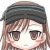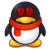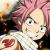## 语法设计，在不使用分号的情况下如何避免下面这种二义性？

```# dof是一个列表
# 第一个元素为一个函数，后面的为参数
# 需要调用第一个元素的函数

dof(0)(dof(1), dof(2), dof(3)) # 获取第一个元素，是函数，调用。

# 以上是最直接的编写方式。
# 但这样有二义性。

# 由于我没有使用分号，不像C/C++可以用;来明确一条语句。
# 所以上面的例子最终会被解析成两条语句：
# dof(0) 函数调用，获取dof的第一个元素
# (dof(1), dof(2), dof(3)) 一个临时列表
#```

1，在dof(0)后面加个.，变成dof(0).(dof(1), dof(2), dof(3))，这样就会被解析成一条语句，在把.()定义为调用函数的另一种形式。或者是.call，这个更明确一点：dof(0).call(dof(1), dof(2), dof(3))

2. 应用一个“不可分”概念，使用[]之类的开合符号作为不可分的单位，例如：[dof(0)(dof(1), dof(2), dof(3))]。这样解析器遇到[]，会将里面的所有表达式视为一个整体的语句，于是不会有二义性了。

```define getf lambda ()
lambda (a b) a + b * 2 end
end

...

getf()(10 20)

...```

1#### 引用来自“艾米”的评论

函数调用差不多应该是 func(p1,p2)

#### 引用来自“句龙胤”的评论

getf()(1, 2)，就产生了两条语句：1.调用函数getf，2.一个临时列表(1, 2)。而不是调用函数getf，然后以(1, 2)为参数调用getf返回的函数。

=============

```define getlist lambda ()
println ("getlist()")
(1, 2, 3)
end

getlist() # result (1, 2, 3)```

```define getf lambda (v)
case v
0    lambda (a, b) a + b end
1    "1"
2    (2)
end
end

x = ...
...
getf(x)
(1, 2)```

=========

C语言里，允许

```typedef int(*test_fn)(int a, int b);

test_fn ret_fn()
{
return (test_fn)1;
}

int c = ret_fn()(1, 2);```

dof(0)(dof(1), dof(2), dof(3)) 为一行时，你应该认为dof(0)返回的是个函数，如果运行时得到的返回不是个函数，你应该报错0函数调用差不多应该是 func(p1,p2)

0#### 引用来自“艾米”的评论

函数调用差不多应该是 func(p1,p2)

getf()(1, 2)，就产生了两条语句：1.调用函数getf，2.一个临时列表(1, 2)。而不是调用函数getf，然后以(1, 2)为参数调用getf返回的函数。

=============

```define getlist lambda ()
println ("getlist()")
(1, 2, 3)
end

getlist() # result (1, 2, 3)```

```define getf lambda (v)
case v
0    lambda (a, b) a + b end
1    "1"
2    (2)
end
end

x = ...
...
getf(x)
(1, 2)```

=========

0`dof(0)(dof(1), dof(2), dof(3))`